# Speed Controlled RPM Matching LED Strip

A Digistump-based LED strip that calculates what RPM corresponds to each gear to aid in heel-toe downshifting and rev-matching. Budget: \$20

IntermediateShowcase (no instructions)12 hours3,079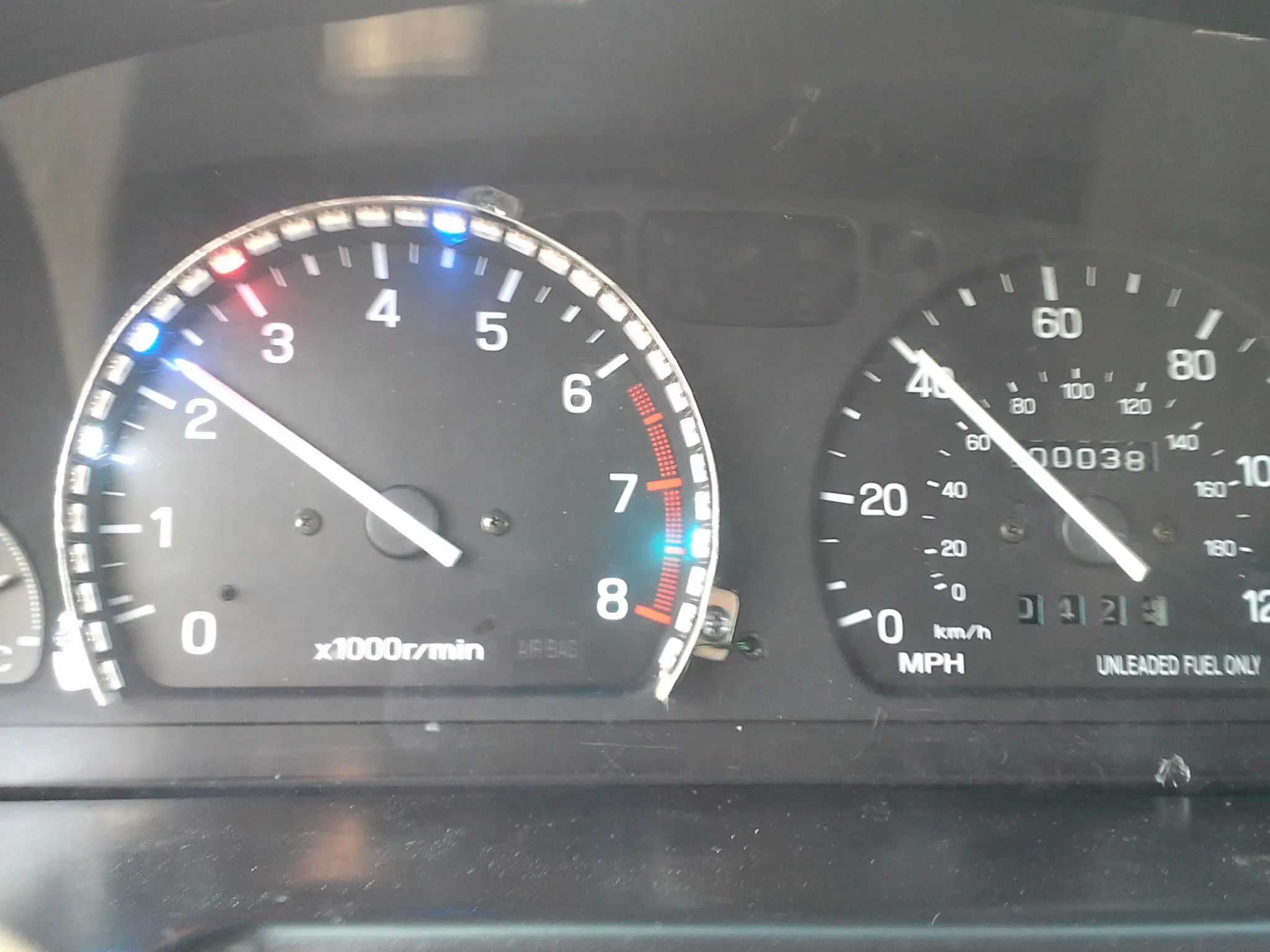## Things used in this project

### Hardware components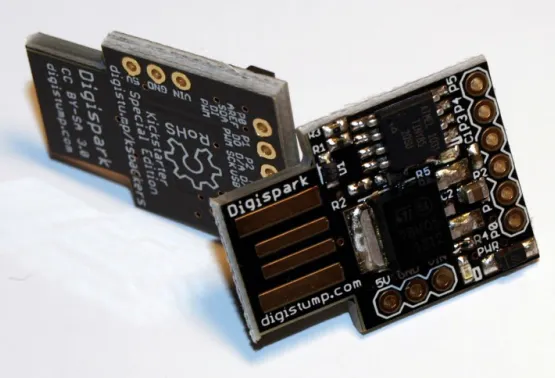DigiSpark
×1
 capacitor 330 uf
×1
 resistor 5k ohm
×2
 WS2812 Strip
×1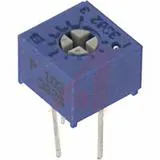Single Turn Potentiometer- 10k ohms
×1Resistor 10k ohm
×1
 Resistor 170 ohm
×1
 IR LED 940nm
×1
 IR Photodiode 940nm
×1
 Resistor 440ohm
×1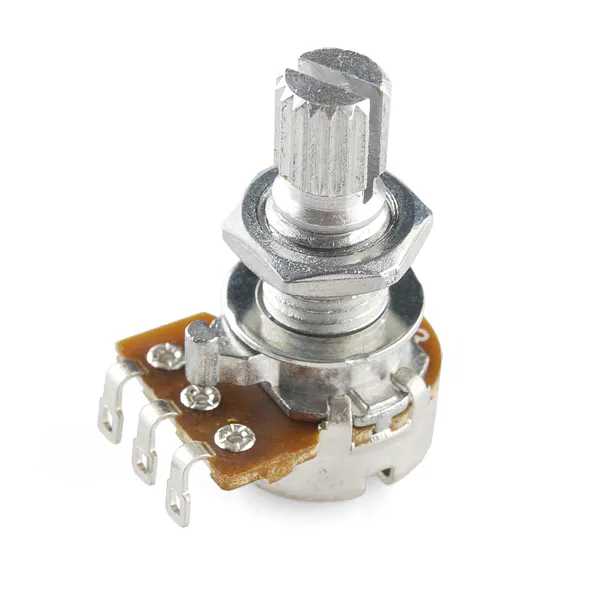Rotary potentiometer (generic) With on/off swtich
×1
 Prototype board
×1
 5v Voltage Regulator
×1

### Software apps and online servicesArduino IDE

## Schematics

### Schematic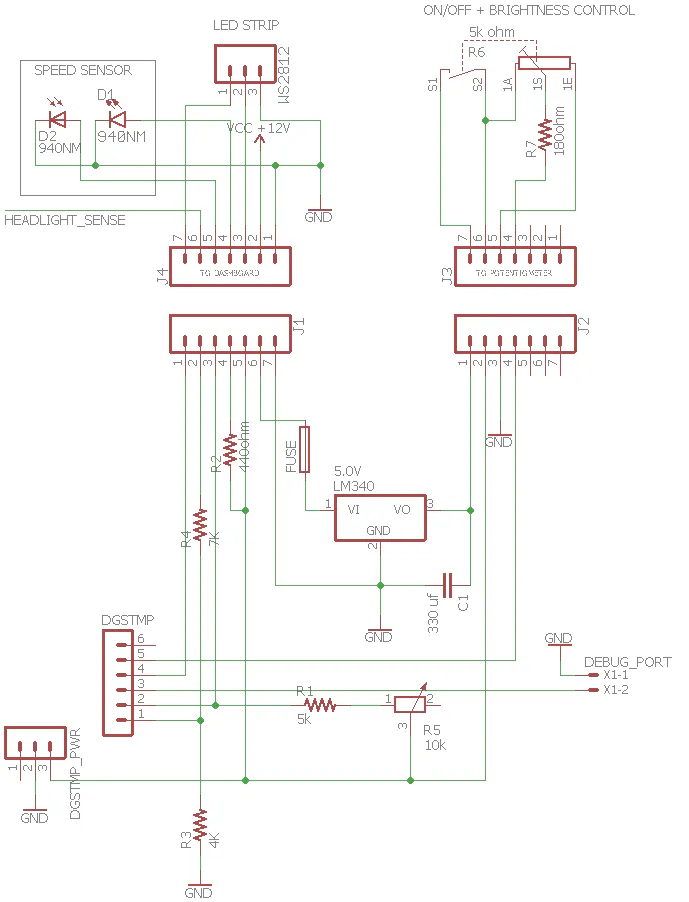## Code

### gear.h

C/C++
```/* This header defines a gear structure allowing for easier-to read
and manipulate code for the NeoPixel gear display car project
*/
#pragma once

struct gear {
public:
double ratio;
uint8_t R;
uint8_t G;
uint8_t B;
gear() {};
gear(double ratio_) {
ratio = ratio_;
}
gear(double ratio_, uint8_t R_, uint8_t G_, uint8_t B_) {
ratio = ratio_;
R = R_;
G = G_;
B = B_;
}
int calcLED(int mph) {
int numLEDs = 31;
double res = (mph - 10 / ratio) / 177.6 * ratio*(numLEDs - 1);  //changed 12 to 10 on 7/29/17
return round(res);
}
};

gear gears;

void init_gears() {
gears = gear(4.842, 0, 200, 200);
gears = gear(2.888, 12, 40, 180);
gears = gear(1.977, 200, 0, 0);
gears = gear(1.519, 32, 12, 255);
gears = gear(1.043, 200, 200, 200);
}
```

### Main code

C/C++
```/*This version works with 8 colored stripes and no night mode (night mode was too problematic)
*Progressive spaces to count based on speed calculation
*Global brightness, global gear variables
*speeds calculation calibrated as of 7/28/17 - spot on
*/
#include "avr/interrupt.h"
#include "gear.h"

//neopixels

//interrupt volatiles
volatile uint32_t count=0;
volatile uint32_t start = 0;
volatile uint32_t finish = 0;
volatile int spaces_to_count = 16;

int display_in_Pixels(double micros_){
//zero out the colors
for (int i = 0; i < 33; i++) { pixels.setPixelColor(i, pixels.Color(0,0,0)); }

//calculate speed in mph
double speeds = 226257.3614/micros_*spaces_to_count;   //newly calibrated as of 7/28/17
spaces_to_count = (speeds)+4;                   //progressive counting:  less to count at low speed = greater refresh rate, but less accurate

for (int i = 0; i < 5; i++){
int pixtoset =  gears[i].calcLED(speeds);
//limit size of pix
if (pixtoset > 31) {pixtoset = 31;}
if (pixtoset < 1) {pixtoset = 0;}
pixels.setPixelColor(32-pixtoset, pixels.Color(gears[i].R, gears[i].G, gears[i].B));
}
}

void setPixBrightness(){
statePin4 = (1023 - statePin4)/8;    //invert
pixels.setBrightness(statePin4);
}

void setup()
{
init_gears();
pixels.begin();
GIMSK = 0b00000000;    // turns off pin change interrupts
PCMSK = 0b00000010;    // turn on interrupts on pins P1
//zero out the colors
for (int i = 0; i < 33; i++) { pixels.setPixelColor(i, pixels.Color(0,0,0)); }
pixels.show();
}

void loop()
{
//reset variables
start = 0;       //going to be the start of the spaces in micros
finish = 0;      //end of the spaces in micros, also flag variable (as in we are done counting)
count = 0;

//re-enable interrupts, and wait for timing to be complete
GIMSK = 0b00100000;    // turns on pin change interrupts
while (!finish){ } // once the right number of spaces have passed, finish is non-zero, so it works as a flag variable

display_in_Pixels(finish-start);

//set brightness
setPixBrightness();

//show pix
pixels.show();
}

ISR(PCINT0_vect)
{
if (!count) {start = micros();}    //if count is 0, the this is the start of the timing
if (count >= spaces_to_count){     //we are done counting
//disable inters
GIMSK = 0b00000000;    // turns off pin change interrupts
finish = micros();     //stop the stopwatch
}
count++;
}
```

## Credits

### Latch Hristov

1 project • 3 followers
Bored, with a cheap hobby.